# CHALLENGE #1: The RiddleA warm-up exercise to get started.
Find the corresponding symbols.
Each line gives a number or a letter.
Hint: It may be a good idea to think out of the (mathematical) box 😉

## SOLUTION: U4W

Proof:

• For the first letter U we write the nominator as AuB; the “u” is originally used as union-symbol. Canceling A and B gives U.

• Second symbol:
Nominator: (2)English = (5)English = Five
Denominator: The first part “T *1.8 + 32” is the translation for a temperature in C° to Fahrenheit, i.e. F, whereas  2.71…. indicates e. Canceling leaves “iv”,“IV” which is 4 in roman numerals.
• Wave – a – v – e = W
a, the acceleration, is the second derivative of the displacement s regarding to the time t.

- beta - eta gives b. The Cyrillic letter b is the synonym for v in Latin letters (https://en.wikipedia.org/wiki/ISO_9)

- 2.71.. = e

# CHALLENGE #2: Finance

A raQoon health technology stock is currently trading at 12 RAQ and the company is releasing the results of a vitamin test. A raQoon-analyst tells you that if the test was successful (which means a healthier raQoon population), the stock will rise to 20 RAQ otherwise it will fall to 8 RAQ.

Assume the stock is perfectly priced according to its future expected value. What is the probability that the vitamin test will be a success?## SOLUTION: 1/3

Proof:

Let S be the RV of the stock price. Then

• E[S(t+1)]=S(t)=12 RAQ (since perfectly priced) and
• E[S(t+1)] = E[S(t+1)|{success}]*P[{success}] + E[S(t+1)|{no success}]*P[{no success}] (simply by calculation rules of conditional expectation)

Hence, combining those two and taking values into account we get

• 12= 20*p + 8*(1-p) where p= P[{success}]
• Therefore p=1/3.

# CHALLENGE #3 ProbabilityLet x be an integer between 1 and 1012.
What is the probability that the cubic of x ends with 11?

## SOLUTION: 0.01 (or 1%)

Proof:

Take any x in {1,...,1012}. Then there are unique a,b in {0,…,9} and c in N0 such that x=a+b*10+c*100

Binomial formula: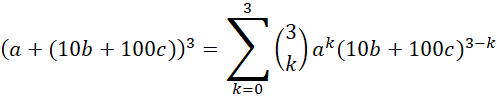By taking mod100 (we are only interested in last two digits) we end up with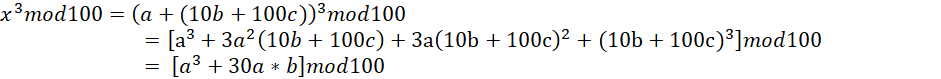Now we want this to be 11: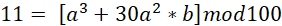Since 30a2b mod10 is always 0, we need a3mod10=1, this is only possible if a=1.

Hence, we end up with the problem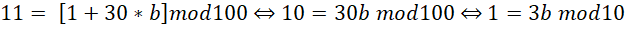Therefore b=7.

So, we conclude that for x3mod100=11 x has to be of the following form: x=100*c+71.

So, exactly 1 in 100 numbers has this property, therefore there is a 1% chance to pick such an x when drawing randomly from a uniform distribution over {1, …, 1012}.

# CHALLENGE #4 RaQoon race

Imagine an equilateral triangle with edges of length 1.85km. At time zero in each corner a raQoon is sitting on a racing-cart.

Now, each raQoon is going with constant velocity 10 km/h in the direction of its right neighbor (i.e. the direction changes all the time). At which point in time will the three racing raQoons crash in the middle of the triangle?

Note: Assume that the raQoon cart has infinite acceleration, i.e. the velocity 10 km/h is already given at time zero.## SOLUTION: 444

Proof:

1. General remark: Take a triangle with edges of uniform length and set the velocity to v.
The problem is symmetric, the raQoons span up an equilateral triangle at each point in time. So without loss of generalization it is enough to have a look only on two raQoons. We have a look on the relative velocity between them: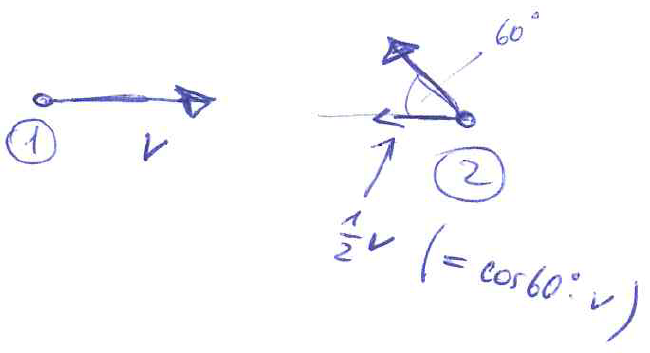2. So the absolute relative velocity between two racoons is 3/2*v. (For a triangle with uniform length this means, the time needed is 2/(3v) (by simply taking 1/(3/2*v).) For our example this means:
1.85 km * 2/(3*10 km/h) = 3.7/30 h =  3.7/30 *602 s = 444s.

# CHALLENGE #5 The Q-Tree

A RaQoon is standing at the bottom of a Q-fruit tree which is 15 metres high and would love to pick Q‘s growing only at the top. Needless to say, the RaQoon starts climbing:

At the beginning of each year, the RaQoon climbs 2cm and then takes a rest of 1 year. In this break, the tree grows by 4cm (uniformly along the whole height). This procedure then repeats year by year. Does our very patient RaQoon reach the top and can harvest the beloved Q‘s? If yes, how many years does it take?

Hints: Imagine the RaQoon as a dot without a chance to grab some Q‘s by reaching out, the hands are needed for climbing of course. Furthermore, assume RaQoons and the Q-fruit tree are getting very, very old. Please answer with either „No“ or with the number of years.